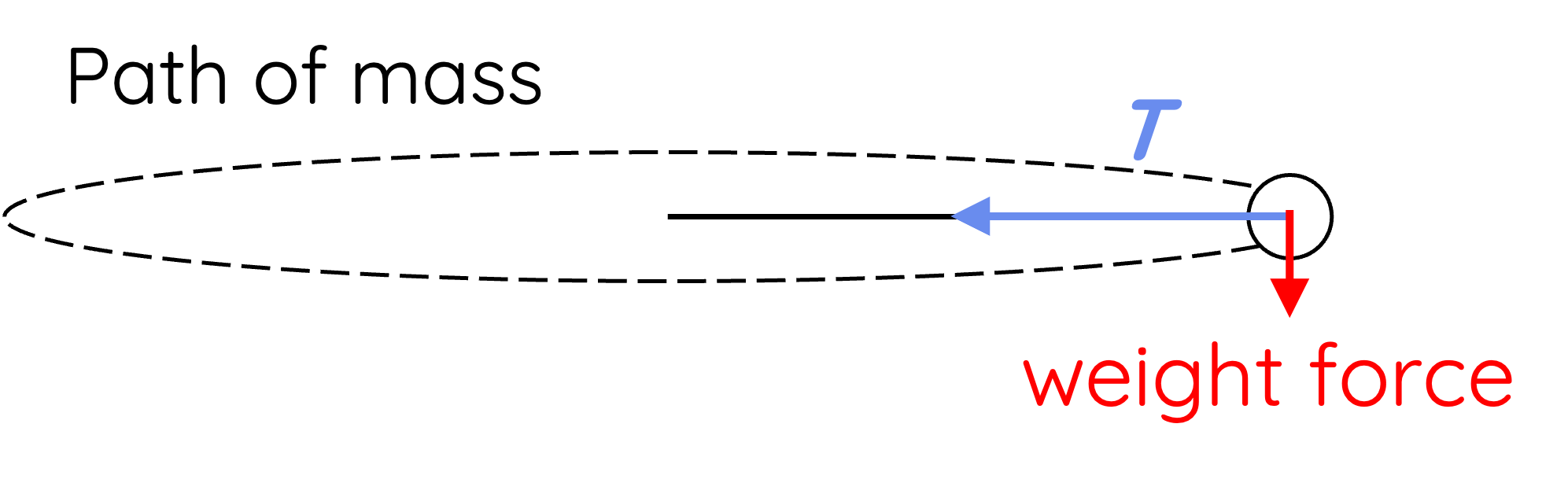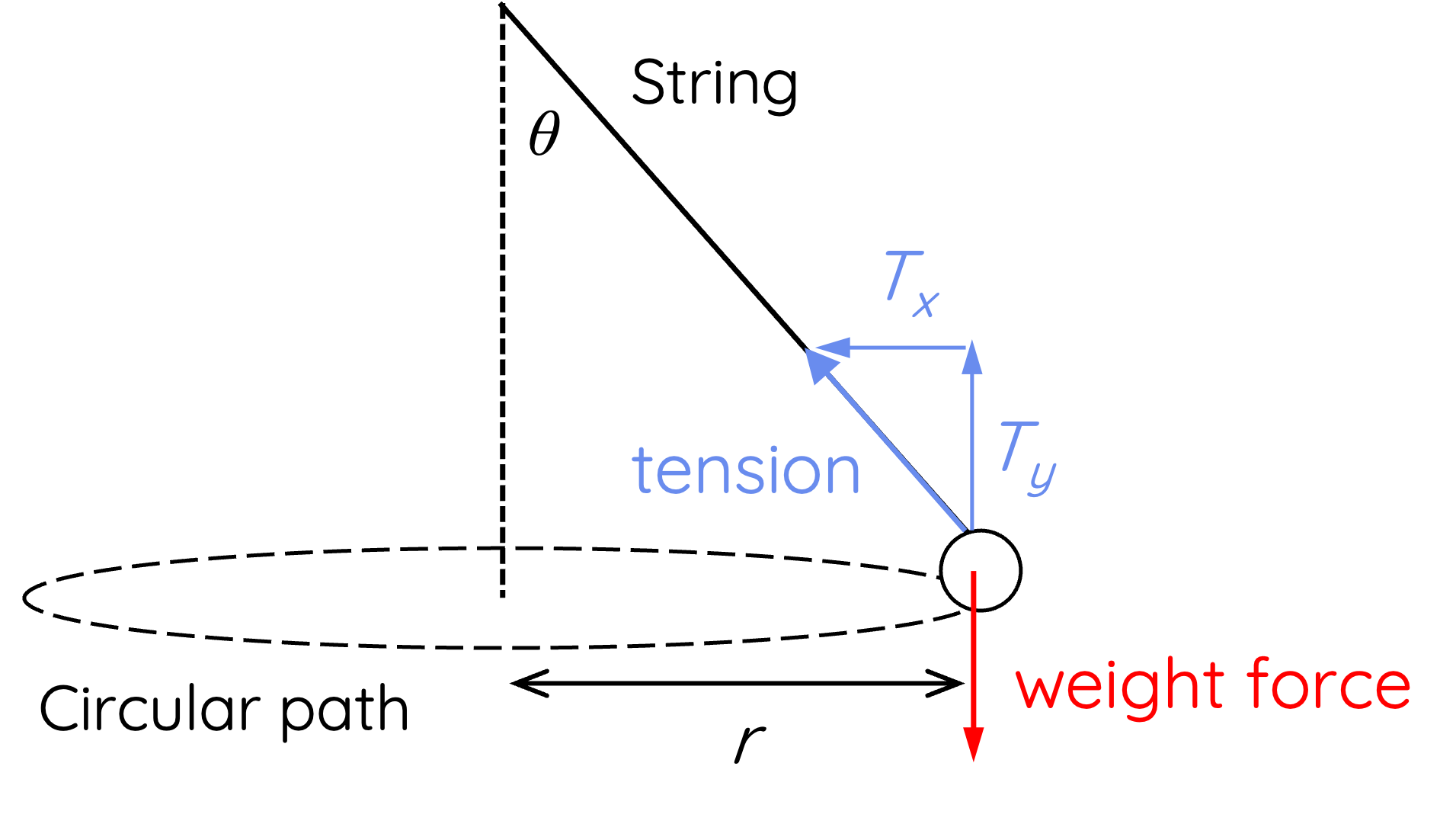# Circular Motion: Mass on a String

This topic is part of the HSC Physics course under the section Circular Motion.

### HSC Physics Syllabus

• analyse the forces acting on an object executing uniform circular motion in a variety of situations, for example:

- cars moving around horizontal circular bends
- a mass on a string
- objects on banked tracks

### Circular Motion Involving Tension Force

This video discusses the analysis of uniform circular motion scenarios involving a mass on a string or rope.

### Understanding Circular Motion with a String

When an object is tethered to a string or rope and set into circular motion, like a ball swung in a circle, it's the tension in the string that provides the necessary centripetal force to keep the object moving in that circle.

### Role of Tension in Circular Motion

Tension is a force exerted by a string or rope when it is stretched. In circular motion with a string:

1. Tension Force: Always pulls inward, towards the centre of the circular path. It contributes to the centripetal force, ensuring the object remains in its circular trajectory.

2. Centripetal Force: This isn’t a new or separate force. Instead, in the context of our spinning object, the tension in the string provides the centripetal force.

The relationship between tension and centripetal force depends on the angle the string or rope makes with either the vertical or horizontal axis.

### When String is Horizontal

Consider a ball or mass attached to a string, undergoing uniform circular motion in a horizontal path as shown.It is important to understand that this situation is only possible if the weight force of the mass is relatively negligible compared to the tension force. This is because the weight force is not balanced by any other forces, and would result in a net force acting downward on the mass, causing the string to create an angle with the horizontal axis.

In this case, the centripetal force is provided by the tension force:

$$F_{\text{centripetal}} = T$$

$$\frac{mv^2}{r} = T$$

This equation suggests that the magnitude of tension force depends on:

• Mass of the object: A heavier object requires more centripetal force, hence more tension, to keep it in a circular path.
• Radius of the circle: A larger circle or greater distance from the centre reduces the tension required, while a smaller circle increases it.
• Speed of the object: The faster the object moves, the greater the necessary centripetal force, and thus the greater the tension in the string.

In order to create such a circular path, the magnitude of tension needs to be substantially greater than the object's weight force. This can be achieved by increasing the object's speed, decreasing the length of the string (thus the radius), or using a smaller mass.

### When String is at an Angle

Consider a ball or mass attached to a string, undergoing uniform circular motion in a horizontal path as shown. The string makes an angle θ with the vertical axis.When the string or rope is at an angle, the direction of the tension force is also at an angle. This means we must resolve the tension force vector into its horizontal and vertical components.

$$T_x = T\sin{\theta}$$

$$T_y = T\cos{\theta}$$

In this case, the only force vector directed towards the centre of the circular path is the horizontal component of tension force. Therefore, the centripetal force is provided by the horizontal component of tension:

$$F_{\text{centripetal}} = T\sin{\theta}$$

$$\frac{mv^2}{r} = T\sin{\theta}$$

The vertical component of tension must balance the downward weight force of the mass if undergoes a horizontal circular motion.

$$mg = T\cos{\theta}$$

By dividing the two equations, we get:

$$\frac{v^2}{rg} = \tan{\theta}$$

Rearranging this equation, we can derive an expression for velocity:

$$v = \sqrt{rg\tan{\theta}}$$

Therefore, the velocity of the mass on a string in circular motion depends on

• acceleration due to gravity
• angle the string makes with the vertical axis; when angle increases, velocity increases

### Vertical Circular Motion

Mass on a string or rope can be swung to create circular motion in a vertical orientation. In this instance, uniform circular motion can be achieved by ensuring that the centripetal force (net force) acting on an object remains constant.

It is important to understand that the direction and magnitude of different forces acting on the mass in a vertical circular motion change depending on its position.For example, while the magnitude and direction of weight force remain unchanged, the direction and magnitude of tension change throughout the motion. At the top of the circular path, tension force acts downward. At the bottom of the circular path, tension force acts upward.

 At the top of the circular motion: -(mv^2)/r=-mg-T_t   T_t=(mv^2)/r-mg At the bottom of the circular motion: (mv^2)/r=-mg+T_b   T_b=(mv^2)/r+mg

When an object attached to a rope is swung vertically, the tension in the rope changes throughout the motion. More tension force is needed at the bottom of the uniform circular motion compared to at the top because gravitational force acts in the opposite direction to centripetal force.